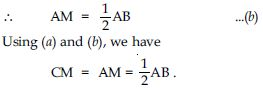# NCERT Solutions for Class 9 Math Chapter 8 - Quadrilaterals

##### Question 1:

The angles of a quadrilateral are in the ratio 3 : 5 : 9 : 13. Find all angles of the quadrilateral.

The angles of a quadrilateral are in the ratio
3 : 5 : 9 : 13.
So, ∠A = 3x
∠B = 5x
∠C = 9x
∠D = 13x
where x is a positive constant.
Now, ∠A + ∠B + ∠C + ∠D = 360$°$
[Angle sum property of a quadrilateral]
$⇒$ 3x + 5x + 9x + 13x = 360$°$
$⇒$ 30x = 360$°$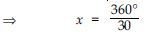$⇒$ x = 12$°$
Now,
∠A = 3x $⇒$ ∠A = 3 × 12$°$ = 36$°$
∠B = 5x $⇒$ ∠B = 5 × 12$°$ = 60$°$
∠C = 9x $⇒$ ∠C = 9 × 12$°$ = 108$°$
and ∠D = 13x $⇒$ ∠D = 13 × 12$°$ = 156$°$
Hence, angles of given quadrilateral are 36$°$, 60$°$, 108$°$ and 156$°$ respectively.

##### Question 2:

If the diagonals of a parallelogram are equal, show that it is a rectangle.

Given: ABCD is a gm with diagonal AC
= diagonal BD
To prove: ABCD is a rectangle.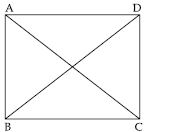Proof: In $∆$s ABC and ABD, we have
AB = AB ... (Common side)
AC = BD (Given)
and AD = BC (Opp. sides of a II gm)
$\therefore$ $∆$ABC $\cong$ $∆$BAD
(By SSS congruence rule)
$⇒$ ∠DAB = ∠CBA ...(i)
(Property of congruency)
But ∠DAB + ∠CBA = 180$°$ ...(ii)
[$\because$ AD II BC and AB cuts them, the sum of the
int. ∠s on the same side of transversal is 180$°$.]
From (i) and (ii), we get:
∠DAB = ∠CBA = 90$°$
Hence, ABCD is a rectangle.
[$\because$ If one angle of II gm is 90$°$, it is a rectangle.]
[Hence Proved]

##### Question 3:

Show that if diagonals of a quadrilateral bisect each other at right angles, then it is a rhombus.

Let ABCD be a rhombus.
Let its diagonal AC and BD bisect each other at right angle at point O.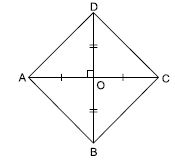$\therefore$ OA = OC, OB = OD
and ∠AOB = ∠BOC = ∠COD
= ∠AOD = 90$°$
We have to prove that ABCD is a rhombus.
In $∆$AOD and $∆$BOC,
OA = OC (Given)
∠AOD = ∠BOC (Each = 90$°$) (Given)
OD = OB (Given)
$\therefore$ $∆$AOD $\cong$ $∆$ COB
(SAS criteria of congruency)
(Corresponding parts of congruent triangles)
In $∆$AOB and $∆$COD,
OA = OC (Given)
∠AOB = ∠COD (Each = 90$°$) (Given)
OB = OD (Given)
$\therefore$ $∆$AOB $\cong$ $∆$COD
(SAS criteria of congruency)
So, AB = CD (Corresponding part of
congruent triangles) ...(ii)
Now in $∆$AOB and $∆$BOC,
AO = OC (Given)
∠AOB = ∠BOC
(each = 90$°$) (Given)
OB = OB (Common)
$\therefore$ $∆$AOB $\cong$ $∆$BOC
(SAS criteria of congruency)
So, AB = BC ...(iii)
(Corresponding parts of congruent triangles)
From (i), (ii) and (iii), we get
AD = BC = CD = AB
So, in addition to given conditions that the diagonals of a quadrilateral bisect each other at right angle; we also have all its sides equal. Hence, quadrilateral satisfies all the conditions to be a rhombus. Therefore given quadrilateral is a rhombus.

##### Question 4:

Show that the diagonals of a square are equal and bisect each other at right angles.

Given: ABCD is a square. AC and BD are its diagonals bisect each other at point O.
To prove: (i) AC = BD, (ii) AC $\perp$ BD at point O.

<img height="218" src= "images/MBD_SR_METH_G9_img_300.jpg" width="360"> Proof: In $∆$s ABC and BAD,
AB = AB (Common side)
∠ABC = ∠BAD (Each 90$°$)
BC = AD (Sides of a square)
$\therefore$ $∆$ABC $\cong$ $∆$BAD [SAS congruence rule]
$⇒$ AC = BD (c.p.c.t.)
Hence, part (i) is proved.
In $∆$s AOB and AOD,
AO = AO (Common sides)
AB = AD (Sides of a square)
OB = OD
(Diagonals of a square bisect each other)
$\therefore$ $∆$AOB $\cong$ $∆$AOD (SSS congruence rule)
$\therefore$ ∠AOB = ∠AOD (c.p.c.t.)
But ∠AOB + ∠AOD = 180$°$ (Linear pair angles)
$\therefore$∠AOB = ∠AOD = 90$°$
i.e. OA $\perp$ BD or AC $\perp$ BD.
Hence, part (ii) is proved.

##### Question 5:

Show that if the diagonals of a quadrilateral are equal and bisect each other at right angles, then it is a square.

Let ABCD be a quadrilateral in which equal diagonals AC and BD bisect each other at right angle at point O.
We have
AC = BD
OA = OC ...(i)
and OB = OD ...(ii)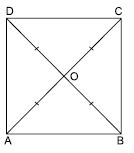AC = BD
$⇒$ OA + OC = OB + OD
$⇒$ OC + OC = OB + OB [Using (i) and (ii)]
$⇒$ 2OC = 2OB
$⇒$ OC = OB ...(iii)
From (i), (ii) and (iii), we get:
OA = OB = OC = OD ...(iv)
Now in $∆$AOB and COD,
OA = OD [Shown in part (iv)]
∠AOB = ∠COD
[Vertically opposite angles]
OB = OC [Shown in part (iv)]
$\therefore$ $∆$AOB $\cong$ $∆$DOC
[SAS criteria of congruency]
So AB = DC (c.p.c.t) ...(v)
Similarly, $∆$BOC $\cong$ $∆$AOD
[SAS criteria of congruency]
So, BC = AD (c.p.c.t) ...(vi)
(v) and (vi) implies that opposite sides of
Hence, ABCD is a parallelogram.
Now in $∆$ABC and $∆$BAD,
AB = BA [Common side]
BC = AD [Proved in part (vi)]
AC = BD (Given)
$\therefore$ $∆$ABC $\cong$ $∆$BAD
[SSS criteria of congruency]
So, ∠ABC = ∠BAD (c.p.c.t) ...(vii)
But ∠ABC + ∠BAD = 180$°$ ...(viii)
[$\because$ ABCD is a parallelogram (Proved above)]
$\therefore$ AD || BC and AB as a transversal
$⇒$ ∠ABC + ∠ABC = 180$°$ [Using (vii) in (viii)]
$⇒$ 2 ∠ABC = 180$°$
$⇒$ ∠ABC = 90$°$
$\therefore$ ∠ABC = ∠BAD = 90$°$ ...(ix)
Opposite angles of a parallelogram are equal
But ∠ABC = 90$°$ and ∠BAD = 90$°$
$\therefore$ ∠ABC = ∠ADC = 90$°$ ...(x)
and ∠BAD = ∠BCD = 90$°$ ...(xi)
We observe that
= ∠BCD = 90$°$ ...(xii)
Now in $∆$AOB and $∆$BOC
OA = OC (given)
∠AOB = ∠BOC [each 90$°$ (given)]
OB = OB (Common)
$\therefore$ $∆$AOB $\cong$ $∆$COB
(SAS rule of congruency)
So, AB = BC ...(xiii)
From (v), (vi) and (xiii), we get:
AB = BC = CD = AD ...(xiv)
Using (xii) and (xiv);
Now we have the quadrilateral whose equal diagonals bisect each other at right angle.
Also sides are equal making an angle of 90$°$ with each other.
Hence, given quadrilateral satisfy all conditions to be a square.

##### Question 6:

Diagonal AC of a parallelogram ABCD bisects ∠A (see fig.). Show that
(i) It bisects ∠C also
(ii) ABCD is a rhombus.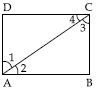It is given that diagonal AC bisects ∠A of the || gm ABCD.
To prove: AC bisects ∠C
Proof: Since AB DC and AC intersects them
$\therefore$ ∠1 = ∠3 (Alternate angles) ...(a)
Similarly, ∠2 = ∠4 ...(b)
But ∠1 = ∠2 ($\because$ AC bisects ∠A) ...(c)
∠3 = ∠4 [Using (a), (b) and (c)]
Thus, AC bisects ∠C.

##### Question 7:

ABCD is a rhombus. Show that the diagonal AC bisects ∠A as well as ∠C and diagonal BD bisects ∠B as well as ∠D.

ABCD is a rhombus.
$\therefore$ AB = BC = CD = AD
Let O be the point of bisection of diagonals.
$\therefore$ OA = OC and OB = OD
In $∆$AOB and $∆$AOD,
OA = OA (Common)
AB = AD[Equal sides of rhombus]
OB = OD
[Diagonals of rhombus bisect each other]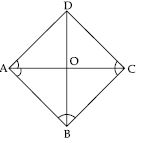$\therefore$ $∆$AOB $\cong$ $∆$AOD
[SSS criteria of congruency]
(Corresponding parts of congruent triangles)
$⇒$ OA bisects ∠A ....(i)
Similarly, $∆$BOC $\cong$ $∆$DOC
(SSS criteria of congruency)
So; ∠OCB = ∠OCD [c.p.c.t]
$⇒$ OC bisects ∠C ...(ii)
From (i) and (ii), we can say that diagonal AC
bisects ∠A and ∠C.
Now in $∆$AOB and $∆$BOC,
OB = OB (Common)
AB = BC [Equal sides of rhombus]
OA = OC
[$\because$ Diagonals of rhombus bisect each other]
$\therefore$ $∆$AOB $\cong$ $∆$COB
[SSS criteria of congruency]
So, ∠OBA = ∠OBC (c.p.c.t)
$⇒$ OB bisects ∠B ...(iii)
Similarly, $∆$AOD $\cong$ $∆$COD [SSS congruency]
$⇒$ ∠ODA = ∠ODC (c.p.c.t.)
From (iii) and (iv) we can say that diagonal BD bisects ∠B and ∠D.

##### Question 8:

ABCD is a rectangle in which diagonal AC bisects ∠A as well as ∠C. Show that:
ABCD is a square

ABCD is a rectangle
$\therefore$ AB = DC ...(a)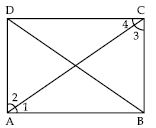Also each angle; ∠A = ∠B = ∠C = ∠D = 90$°$
In $∆$ABC and $∆$ADC,
∠1 = ∠2
and ∠3 = ∠4
[$\because$ AC bisects ∠A and ∠C (given)]
AC = AC (Common)
$\therefore$ $∆$ABC $\cong$ $∆$ADC
(ASA criteria of congruency)
From (a) and (b), we get:
AB = BC = AD = DC
It implies that all sides of a rectangle are
equal.
Hence, it is a square.

##### Question 9:

ABCD is a rectangle in which diagonal AC bisects ∠A as well as ∠C. Show that:
Diagonal BD bisects both ∠B as well as ∠D.

ABCD is a rectangle
$\therefore$ AB = DC ...(a)Also each angle; ∠A = ∠B = ∠C = ∠D = 90$°$
In $∆$ABD and $∆$BDC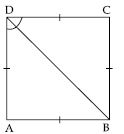AB = BC [$\because$ Rectangle ABCD is a square proved in part (i)]
(proved in part (i) as ABCD is a square)
BD = BD (Common)
$\therefore$ $∆$ABD $\cong$ $∆$CBD
(SSS criteria of congruency)
So, ∠ABD = ∠CBD (c.p.c.t.) ...(c)
and ∠ADB = ∠CDB (c.p.c.t.) ...(d)
(c) and (d) implies that diagonal BD bisects
both ∠B and ∠D.

##### Question 10:

In parallelogram ABCD, two points P and Q are taken on diagonal BD such that DP = BQ (see fig.). Show that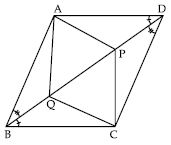$∆$APD $\cong$ $∆$CQB

In $∆$APD and $∆$CQB,
DP = BQ (given)
[$\because$ In parallelogram ABCD,
AD BC; BD being a transversal.
$\therefore$ ∠ADB = ∠DBC (alternate angles)
AD = CB [$\because$ Opposite sides of parallelogram are equal]
$\therefore$ $∆$APD $\cong$ $∆$CQB (SAS criteria of congruency)

##### Question 11:

In parallelogram ABCD, two points P and Q are taken on diagonal BD such that DP = BQ (see fig.). Show thatAP = CQ

So; AP = CQ (Corresponding parts of congruent triangles)

##### Question 12:

In parallelogram ABCD, two points P and Q are taken on diagonal BD such that DP = BQ (see fig.). Show that$∆$AQB $\cong$ $∆$CPD

In $∆$AQB and $∆$CPD,
BQ = DP (Given)
∠ABQ = ∠PDC
[$\because$ In parallelogram ABCD,
AB || CD; BD being a transversal.
$\therefore$ ∠ABD = ∠BDC (Alternate angles)
So;∠ABQ = ∠PDC]
AB = CD [$\because$Opposite sides of a parallelogram are equal]
$\therefore$ $∆$AQB $\cong$ $∆$CPD
[SAS criteria of congruency]

##### Question 13:

In parallelogram ABCD, two points P and Q are taken on diagonal BD such that DP = BQ (see fig.). Show thatAQ = CP

So, AQ = CP (Corresponding parts of congruent triangles)

##### Question 14:

In parallelogram ABCD, two points P and Q are taken on diagonal BD such that DP = BQ (see fig.). Show thatAPCQ is a parallelogram

AP = CQ [Proved in part (ii)]
AQ = CP [Proved in part (iv)]
Opposite sides of quadrilateral APCQ are equal.
As we know that in a parallelogram opposite sides are equal.
Hence, APCQ is a parallelogram.

##### Question 15:

ABCD is a parallelogram and AP and CQ are the perpendiculars from vertices A and C on its diagonal BD (see fig.).
Show that: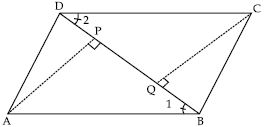$∆$APB $\cong$ $∆$CQD

ABCD is a parallelogram
$\therefore$ AB DC
BD being a transversal.
So, ∠1 = ∠2 (Alternate angles)
Now in $∆$APB and $∆$CQD,
∠APB = ∠CQD (each = 90$°$) (Given)
∠1 = ∠2 (Proved above)
AB = CD [$\because$Opposite sides of a
parallelogram are equal]
$\therefore$ $∆$APB $\cong$ $∆$CQD
(AAS criteria of congruency)

##### Question 16:

ABCD is a parallelogram and AP and CQ are the perpendiculars from vertices A and C on its diagonal BD (see fig.).
Show that:AP = CQ

ABCD is a parallelogram
$\therefore$ AB DC
BD being a transversal.
So, ∠1 = ∠2 (Alternate angles)
So, AP = CQ [Corresponding parts of congruent triangles]

##### Question 17:

In $∆$ABC and $∆$DEF; AB = DE, AB II DE, BC = EF and BC II EF. Vertices A, B and C are joined to vertices D, E and F respectively (see fig.).
Show that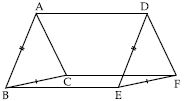Given: In $∆$ABC and $∆$DEF,
AB = DE and AB II DE
Also in $∆$s, BC = EF and BC II EF
In quad. ABED, its one pair of opposite sides AB and DE are such that AB = DE and AB II DE
$\therefore$ ABED is a II gm.
$\therefore$ AD = BE and AD II BE.
[Opp. sides of a gm are equal and parallel] ... (i)

##### Question 18:

In $∆$ABC and $∆$DEF; AB = DE, AB II DE, BC = EF and BC II EF. Vertices A, B and C are joined to vertices D, E and F respectively (see fig.).
Show thatGiven: In $∆$ABC and $∆$DEF,
AB = DE and AB II DE
Also in $∆$s, BC = EF and BC II EF
BE = CF and BE CF
$\therefore$ BEFC is a gm.

##### Question 19:

In $∆$ABC and $∆$DEF; AB = DE, AB DE, BC = EF and BC EF. Vertices A, B and C are joined to vertices D, E and F respectively (see fig.).
Show thatGiven: In $∆$ABC and $∆$DEF,
AB = DE and AB II DE
Also in $∆$s, BC = EF and BC EF
$\therefore$ CF = BE and CF II BE ... (ii)
From (1) and (2), we get

##### Question 20:

In $∆$ABC and $∆$DEF; AB = DE, AB II DE, BC = EF and BC II EF. Vertices A, B and C are joined to vertices D, E and F respectively (see fig.).
Show thatGiven: In $∆$ABC and $∆$DEF,
AB = DE and AB II DE
Also in $∆$s, BC = EF and BC II EF
$\therefore$ ACFD is a IIgm. [$\because$If one pair of opp. sides
of quad. is equal and parallel, it is a IIgm.]

##### Question 21:

In $∆$ABC and $∆$DEF; AB = DE, AB II DE, BC = EF and BC II EF. Vertices A, B and C are joined to vertices D, E and F respectively (see fig.).
Show thatAC = DF

Given: In $∆$ABC and $∆$DEF,
AB = DE and AB II DE
Also in $∆$s, BC = EF and BC II EF
Hence, AC = DF [Opp. sides of a gm]

##### Question 22:

In $∆$ABC and $∆$DEF; AB = DE, AB II DE, BC = EF and BC II EF. Vertices A, B and C are joined to vertices D, E and F respectively (see fig.).

Show that$∆$ABC $\cong$ $∆$DEF

Given: In $∆$ABC and $∆$DEF,
AB = DE and AB II DE
Also in $∆$s, BC = EF and BC II EF
In $∆$ABC and $∆$DEF,
AB = DE (Given)
BC = EF (Given)
AC = DF [Proved in part (v)]
$\therefore$ $∆$ABC $\cong$ $∆$DEF
(SSS criteria of congruency)

##### Question 23:

ABCD is a trapezium in which AB II CD and AD = BC (See fig.)
Show that:
∠A = ∠B

Extend AB and draw a line CE parallel to AD as shown in the figure.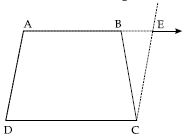Since, AD II CE and transversal AE cuts them at A and E respectively.
$\therefore$ ∠A + ∠E = 180$°$
$⇒$ ∠A = 180$°$ – ∠E ...(i)
Since AB II CD and AD II CE
$\therefore$ AECD is a IIgm
$⇒$ AD = CE
$⇒$ BC = CE [$\because$AD = BC (Given)]
Thus, in $∆$BCE, we have
BC = CE
$⇒$ ∠CBE = ∠CEB
[Equal angles opp. to equal sides]
$⇒$ 180$°$ – ∠B = ∠E
[$\because$ ∠CBE + ∠ABC = 180$°$ (Linear pair)
$\therefore$ ∠CBE = 180$°$ – ∠ABC]
$⇒$ 180$°$ – ∠E = ∠B ...(ii)
From (i) and (ii), we get ∠A = ∠B

##### Question 24:

ABCD is a trapezium in which AB CD and AD = BC (See fig.)
Show that:
∠C = ∠D

ABCD is a trapezium in which AB || DC
$\therefore$ ∠A + ∠D = 180$°$ ...(a)
and ∠B + ∠C = 180$°$ ...(b)
[$\because$For two parallel lines sum of interior angles on the same side of a transversal is 180$°$.]
Equating (a) and (b), we get:
∠A + ∠D = ∠B + ∠C
But ∠A = ∠B proved in part (i)
$\therefore$ ∠A + ∠D = ∠A + ∠C
$⇒$ ∠D = ∠C
or ∠C = ∠D [Hence proved]

##### Question 25:

ABCD is a trapezium in which AB CD and AD = BC (See fig.)
Show that:
$∆$ABC $\cong$ $∆$BAD

In $∆$ABC and $∆$BAD,
AB = AB, (Common)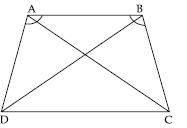∠A = ∠B [Proved in part (i)]
$\therefore$ $∆$ABC $\cong$ $∆$BAD
(SAS criteria of congruency)

##### Question 26:

ABCD is a trapezium in which AB CD and AD = BC (See fig.)
Show that:
diagonal AC = diagonal BD.

So, AC = BD (Corresponding part of congruent triangles)
i.e., in trapezium ABCD;
diagonal AC = diagonal BD.

##### Question 27:

ABCD is a quadrilateral in which P, Q, R and S are the mid-points of sides AB, BC, CD and DA respectively (see fig.)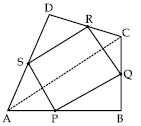AC is a diagonal.
Show that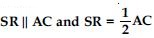In $∆$ABC,
P is the mid-point of AB and Q is the mid-point of BC.
Then PQ II AC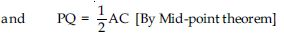In $∆$ACD,
R is the mid-point of CD and S is the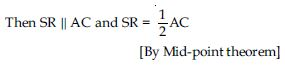##### Question 28:

ABCD is a quadrilateral in which P, Q, R and S are the mid-points of sides AB, BC, CD and DA respectively (see fig.)AC is a diagonal.
Show that
PQ = SR

In $∆$ABC,
P is the mid-point of AB and Q is the mid-point of BC.
Then PQ AC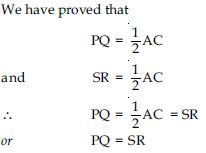##### Question 29:

ABCD is a quadrilateral in which P, Q, R and S are the mid-points of sides AB, BC, CD and DA respectively (see fig.)AC is a diagonal.
Show that
PQRS is a parallelogram.

In $∆$ABC,
P is the mid-point of AB and Q is the mid-point of BC.
Then PQ II ACWe have proved that
PQ II AC
and SR II AC
$⇒$ PQ II SR [$\because$ Two lines parallel
to a given line are parallel to each other.]
Now, we have
PQ = SR
and PQ II SR
As we know that if one pair of opposite sides of a quadrilateral is equal and parallel then it is a parallelogram.
$\therefore$ PQRS is a parallelogram.

##### Question 30:

ABCD is a rhombus and P, Q, R, S are the mid-points of AB, BC, CD and DA respectively. Prove that quadrilateral PQRS is a rectangle.

Given: P, Q, R and S are the mid-points of respective sides AB, BC, CD and DA of rhombus.
PQ, QR, RS and SP are joined.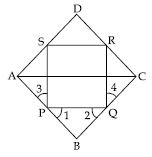To prove: PQRS is a rectangle.
Construction: Join A and C.
Proof: In $∆$ABC, P is the mid-point of AB and Q is the mid-point of BC.
$\therefore$ By mid-point theorem,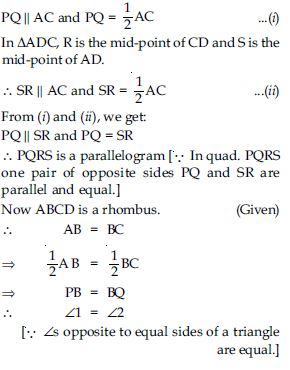Now in $∆$s APS and CQR, we have AP = CQ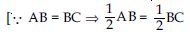$⇒$ AP = CQ where P and Q are midpoints
of AB and BC.]
Similarly, AS = CR
PS = QR
[Opposite sides of gm PQRS]
$\therefore$ $∆$APS $\cong$ $∆$CQR
[By SSS Congruence rule]
$\therefore$ ∠3 = ∠4 (c.p.c.t.)
Now we have
∠1 + ∠SPQ + ∠3 = 180$°$
and ∠2 + ∠PQR + ∠4 = 180$°$ [Linear pairs]
$\therefore$ ∠1 + ∠SPQ + ∠3 = ∠2 + ∠PQR + ∠4
Since ∠1 = ∠2 and ∠3 = ∠4
(As proved above)
$\therefore$ ∠SPQ = ∠PQR ... (iii)
Now, PQRS is a gm (as proved above)
$\therefore$ ∠SPQ + ∠PQR = 180$°$ ... (iv)
[$\because$ SP RQ and PQ cuts them and the sum
of int. ∠s on the same side of a transversal is
180$°$.]
Using (iii) in (iv) we get:
∠SPQ + ∠SPQ = 180$°$
$⇒$ 2∠SPQ = 180$°$
$⇒$ ∠SPQ = 90$°$
Thus, PQRS is a gm whose one angle
∠SPQ = 90$°$.
Hence, PQRS is a rectangle.

##### Question 31:

ABCD is a rectangle and P, Q, R and S are the mid-points of the sides AB, BC, CD and DA respectively. Show that the quadrilateral PQRS is a rhombus.

Given: A rectangle ABCD in which P, Q, R and S are the mid-points of sides AB, BC, CD and DA respectively PQ, QR, RS and SP are joined.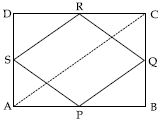To prove: PQRS is a rhombus
Construction: Join AC
Proof: In $∆$ABC, P and Q are the mid-points of sides AB, BC respectively.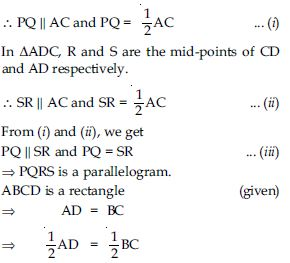$⇒$ AS = BQ ... (iv)
In $∆$s APS and BPQ, we have:
AP = BP
[$\because$ P is the mid-point of AB.]
∠PAS = ∠PBQ [Each is 90$°$]
and AS = BQ [From (iv)]
$\therefore$ $∆$APS $\cong$ $∆$BPQ
[SAS criterion of congruency]
$⇒$ PS = PQ ... (v)
[Corresponding parts of congruent triangles are equal]
From (iii) and (v), we get PQRS is a
parallelogram such that
PS = PQ
i.e., two adjacent sides are equal.
Hence, PQRS is a rhombus.

##### Question 32:

In a parallelogram ABCD, E and F are the mid-points of sides AB and CD respectively (see fig.). Show that the line segments AF and EC trisect the diagonal BD.

Since E and F are the mid-points of AB and CD respectively.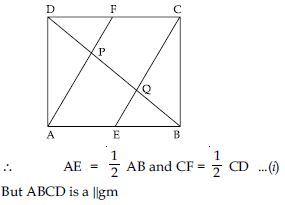$⇒$ AB = CD and AB DC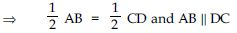$⇒$ AE = FC and AE II FC [From (i)]
$⇒$ AECF is a IIgm
$⇒$ FA II CE
$⇒$ FP II CQ ...(ii)
[$\because$ FP is a part of FA and CQ is a part of CE]
We know that, the segment drawn through the mid-point of one side of a triangle and parallel to the other side bisects the third side.
In $∆$DCQ, F is the mid-point of CD and
FP II CQ [From (ii)]
$\therefore$ P is the mid-point of DQ
$⇒$ DP = PQ ...(iii)
Similarly, in $∆$ABP, E is the mid-point of AB
and EQ II AP
$\therefore$ Q is the mid-point of BP
$⇒$ BQ = PQ ...(iv)
From (iii) and (iv), we have
DP = PQ = BQ ...(v)
Now BD = BQ + PQ + DP
= BQ + BQ + BQ
$⇒$ BD = 3BQ
or 3BQ = BD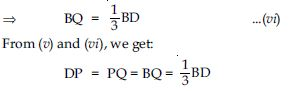It implies that points P and Q trisects BD.
So, AF and CE trisects BD.

##### Question 33:

Show that the line segments joining the midpoints of opposite sides of a quadrilateral bisect each other.

A quadrilateral ABCD in which EG and FH are the line-segments joining the mid-points of opposite sides of a quadrilateral.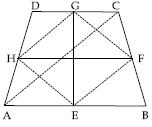To Prove: EG and FH bisect each other.
Construction: Join AC, EF, FG, GH and HE
Proof: In $∆$ABC, E and F are the mid-points of respective sides AB and BC.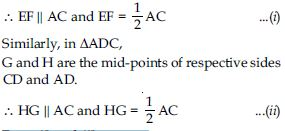From (i) and (ii), we get:
EF HG and EF = HG
$\therefore$ EFGH is a parallelogram.
[$\because$ A quadrilateral is a parallelogram if one pair of its opposite sides is equal and parallel.]
As we know, that the diagonals of a parallelogram bisect each other, therefore line segments (i.e. diagonals) EG and FH (of parallelogram EFGH) bisect each other.

##### Question 34:

ABC is a triangle right angled at C. A line through the mid-point M of hypotenuse AB and parallel to BC intersects AC at D.
Show that:
D is the mid-point of AC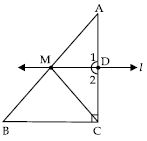In $∆$ABC, M is the mid-point of AB. (Given) MD II BC $\therefore$ AD = DC[Converse of Mid-point theorem] Thus, D is the mid-point of AC.

##### Question 35:

ABC is a triangle right angled at C. A line through the mid-point M of hypotenuse AB and parallel to BC intersects AC at D.
Show that:
MD $\perp$ ACl II BC (Given)
Consider AC as a transversal
$\therefore$ ∠1 = ∠C(Corresponding angles)
$⇒$ ∠1 = 90$°$ [$\because$ ∠C = 90$°$ (Given)]
Thus, MD $\perp$ AC.

##### Question 36:

ABC is a triangle right angled at C. A line through the mid-point M of hypotenuse AB and parallel to BC intersects AC at D.
Show that: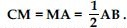In $∆$AMD and $∆$CMD,
∠1 = ∠2 (Each = 90$°$)
$∆$AMD $\cong$ $∆$CMD